Worksheets and No Prep Teaching Resources
Critical Thinking Puzzles
Make Puzzles

# Equation Unknown Puzzles Puzzle Workbooks for Kids - Updated Each Month!

Equation Unknowns puzzles are another great way to teach critical thinking skills while also drilling math skills. Given values are represented by shapes, from which students solve equations and deduce the values. Again, there are multiple puzzle types, and you can choose from various customization options. At their easiest levels, Equation Unknowns puzzles involve addition or subtraction and only two shapes, but as the puzzles increase in difficulty, they can include three and four shapes, a mix of operations, and negative numbers. If your students just can't get enough, you can even print one page of each puzzle, resulting in thirty-eight pages of puzzles!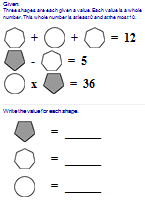Equation Unknowns Book - All of the Puzzles!
One page of each puzzle (38 total pages)

Very Easy
Look at the greater and less than clues. One of the values will be obvious.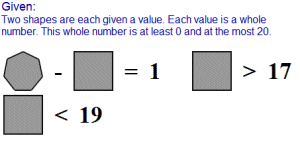addition; 2 shapes subtraction; 2 shapes addition or subtraction; 2 shapes One page of each puzzle

Easy - 2 Shapes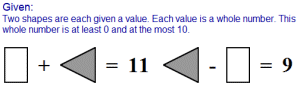2 shapes; numbers 0 to 10; addition 2 shapes; numbers 0 to 10; subtraction 2 shapes; numbers 0 to 10; addition and subtraction 2 shapes; numbers 0 to 10; multiplication 2 shapes; numbers 0 to 10; division One page of each puzzle

3 Shapes - Includes 1 Redundant Rule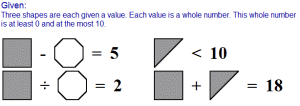3 shapes; numbers 0 to 10; 1 redundant rule; addition 3 shapes; numbers 0 to 10; 1 redundant rule; subtraction 3 shapes; numbers 0 to 10; 1 redundant rule; addition and subtraction 3 shapes; numbers 0 to 10; 1 redundant rule; multiplication 3 shapes; numbers 0 to 10; 1 redundant rule; division One page of each puzzle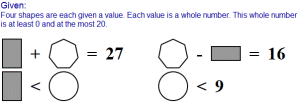3 shapes; numbers 0 to 20; addition and subtraction 4 shapes; numbers 0 to 20; addition and subtraction 5 shapes; numbers 0 to 20; addition and subtraction One page of each puzzle

Mix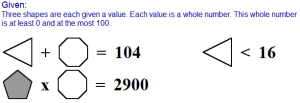3 shapes; numbers 0 to 50 (no division) 3 shapes; numbers 0 to 50 (division included) 4 shapes; numbers 0 to 50 (no division) 4 shapes; numbers 0 to 50 (division included) 5 shapes; numbers 0 to 50 3 shapes; numbers 0 to 100 4 shapes; numbers 0 to 100 One page of each puzzle

Equations with Three Unknowns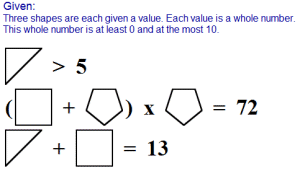no parenthesis (3 shapes) no parenthesis (4 shapes) includes parenthesis (3 shapes) includes parenthesis (4 shapes) One page of each puzzle

Equations with Three Unknowns; Larger Numbers (2 redundant rules to make easier to solve)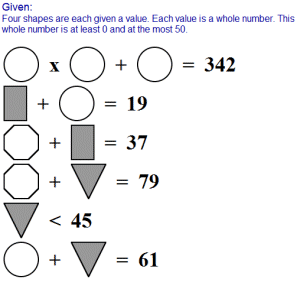3 shapes 4 shapes One page of each puzzle

Negative Numbers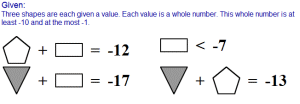2 shapes; addition 2 shapes; subtraction 2 shapes; multiplication 2 shapes; division 3 shapes; addition 3 shapes; subtraction 3 shapes; multiplication 3 shapes; division 3 shapes; mix of operations One page of each puzzle

Have a suggestion or would like to leave feedback?